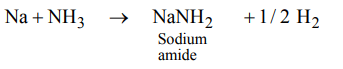## The s-Block Elements Questions and Answers Part-7

1. Which of the following statements is incorrect?
a) Pure sodium metal dissolves in liquid ammonia to give blue solution
b) NaOH reacts with glass to give sodium silicate
c) Aluminium reacts with excess NaOH to give $Al\left(OH\right)_{3}$
d) $NaHCO_{3}$  on heating gives $Na_{2}CO_{3}$

Explanation:2. The ease of adsorption of the hydrated alkali metal ions on an ion-exchange resins follows the order :
a) $Li^{+}<K^{+}<Na^{+}<Rb^{+}$
b) $Rb^{+}<K^{+}<Na^{+}<Li^{+}$
c) $K^{+}<Na^{+}<Rb^{+}<Li^{+}$
d) $Na^{+}<Li^{+}<K^{+}<Rb^{+}$

Explanation:3. $KO_{2}$ (potassium super oxide) is used in oxygen cylinders in space and submarines because it
a) absorbs $CO_{2}$ and increases $O_{2}$ content
b) eliminates moisture
c) absorbs $CO_{2}$
d) produces ozone

Explanation:4. The ionic mobility of alkali metal ions in aqueous solution is maximum for
a) $Li^{+}$
b) $Na^{+}$
c) $K^{+}$
d) $Rb^{+}$

Explanation:5. The products obtained on heating $LiNO_{2}$ will be
a) $Li_{2}O+NO_{2}+O_{2}$
b) $Li_{3}N+O_{2}$
c) $Li_{2}O+NO+O_{2}$
d) $LiNO_{3}+O_{2}$

Explanation:6. What is the best description of the change that occurs when $Na_{2}O\left(s\right)$   is dissolved in water ?
a) Oxide ion accepts sharing in a pair of electrons
b) Oxide ion donates a pair of electrons
c) Oxidation number of oxygen increases
d) Oxidation number of sodium decreases

Explanation: Oxide ion donates a pair of electrons

7. On heating sodium metal in a current of dry ammonia gas the compound formed is
a) sodium nitrate
b) sodium hydride
c) sodium amide
d) sodium azide

Explanation:8. The pair of compounds which cannot exits together in solution is
a) $NaHCO_{3}$  and NaOH
b) $Na_{2}CO_{3}$  and $NaHCO_{3}$
c) $Na_{2}CO_{3}$  + NaOH
d) $NaHCO_{3}$  and NaCl

Explanation:9. Gun powder is
a) $KNO_{3}+Charcoal+S$
b) $NaNO_{3}+KNO_{3}+S$
c) $NaNO_{3}+S$
d) None of these

a) $CO_{2}$  and $NH_{3}$
b) $CO_{2}$  and $NH_{4}Cl$
d) $CaCl_{2}$  and CaO